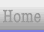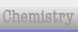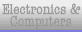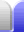# Sequences: Matchsticks

### Overview

Matchsticks are often used to illustrate sequences because matches can be used to make patterns that build in easily predictable ways.

The formula for the matchstick sequence below is quite simple, and can be worked out straight away after counting the first few sequence terms, but how do we show that it really is the formula? We can figure it out two ways.

### Method 1

Work out a formula for the number of horizontal matches and multiply by 2, since the number of vertical matches is the same and the number of horizontal matches.

Calculating the first few terms of the sequence shows some patterns, such as multiplying the number of squares across, or n + 1, by 4, the number of complete rows of matches. However, the first term doesn't fit that rule, since it doesn't have four rows of matches. The answer lies in the number of extra matches (shown in red), which is a sequence within our sequence. It increases by two for each value of n. and for the first term (ie, when n = 1) it has a negative value: -2, 0, 2, 4, 6, ..., 2(n-2).

### Method 2

Work out how many matches are being added each time, treating the figure as a chain of squares.

The basic figure is a chain of squares, just in a loop instead of a straight line. Alternatively, think of it as four individual chains, each touching the next one at a right angle. A straight chain of matchstick squares has the equation 3n + 1 because three matches are added each term, and the original figure (where n = 1) has four matches, not just three. For our loop chain of squares, we'll have one square, or three matches, added to each side each time. The end of each chain doesn't have the extra match needed for a straight chain because the next chain over provides it.

Thinking about that for a moment, because each chain of squares starts off adjoining the next chain over, the first term in the sequence has four squares of three matches each, each using the square next to them to complete their shape. So we don't need to add any extra matches, even for the first term in the sequence.

So the increase in each term in the sequence will be 4 sides (of the figure) x 3 matches, or 12 matches each term. Generically, 4 sides x n squares x 3 matches, or 12n matches. So we know our formula will be 12n. (BTW, this means the "zeroth" term will have no matches.)

### Table

 n diagram matchstick count method 1 working formula check 1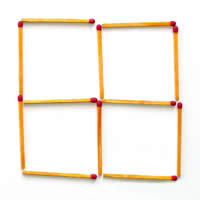horizontal: 2 matches in each of 3 rows vertical: same as horizontal 2 x (2 x 3) = 2 x (2 x 4 - 2) = 2 x 6 = 12 12n 12 x 1 = 122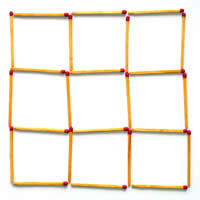horizontal: 3 matches in each of 4 rows vertical: same as horizontal 2 x (3 x 4 + 0) = 2 x 12 = 24 12n 12 x 2 = 243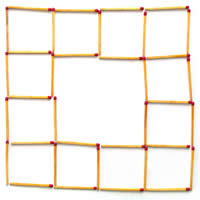horizontal: 4 matches in each of 4 rows plus 2 extras vertical: same as horizontal 2 x (4 x 4 + 2) 2 x (16 + 2) = 2 x 18 = 36 12n 12 x 3 = 364horizontal: 5 matches in each of 4 rows plus 4 extras vertical: same as horizontal 2 x (5 x 4 + 4) = 2 x (20 + 4) = 2 x 24 = 48 12n 12 x 4 = 485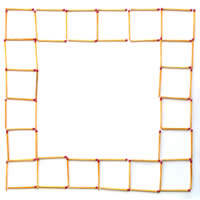horizontal: 6 matches in each of 4 rows plus 6 extras vertical: same as horizontal 2 x (6 x 4 + 6) = 2 x (24 + 6) = 2 x 30 = 60 12n 12 x 5 = 60n horizontal: (n+1) matches in each of 4 rows plus 2(n-2) extras vertical: same as horizontal 2 x ( 4(n+1) + 2(n-2) ) 2 x (4n+4 + 2n-4) 2 x (6n) 12n 12n in a sense, method 2 is the check here 50(To count the matches, click for a larger version.) horizontal: 51 matches in each of 4 rows plus lots extras vertical: same as horizontal 12 x 50 = 600 12n method 2, count the squares: 4 rows (or columns) of 50 squares x 3 matches each 4 x 50 x 3 = 600Home Astronomy Chemistry Electronics & Computers Mathematics Physics Field Trips Turn on javascript for email link